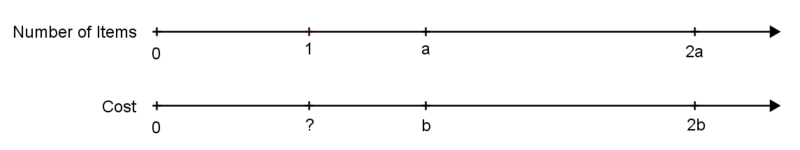# Equivalent Ratios and Unit Rates

Alignments to Content Standards: 6.RP.A.2

2 bottles of water cost $5.00. 1. Fill in the table that shows the costs for 4, 6, and 8 bottles. Find the cost for a single bottle in each case. Number of bottles Cost (\$) Cost per bottle
$$2$$ $$5$$
$$4$$
$$6$$
$$8$$

5 granola bars cost $4.00 1. Fill in the table that shows the costs for 10, 15, and 20 granola bars. Find the cost for a single granola bar in each case. Number of granola bars Cost (\$) Cost per bar
$$5$$ $$4$$
$$10$$
$$15$$
$$20$$

1. Explain why if you can buy $a$ items for $b$ dollars, or buy $2a$ items for $2b$ dollars, the cost per item is the same in either case.

## IM Commentary

This task should come after students have done extensive work with representing equivalent ratios and understand that for any ratio $a:b$, the ratio $sa:sb$ is equivalent to it for any $s>0$. The purpose of this task is to make explicit the fact that equivalent ratios have the same unit rate. A solid understanding of this fact will allow students to solve problems involving equivalent ratios in a very efficient manner. For example, consider the problem "7 identical, full containers hold 4 gallons of water. How many of these containers would you need for 18 gallons?" Once you know that all equivalent ratios have the same unit rate, you could approach this problem with using a very abbreviated ratio table:

 containers gallons 7 1.75 31.5 4 1 18

To compute the unit rates in the task, students should be encouraged to use any representation that makes sense to them.

The abstract nature of part (c) may pose a challenge and gives students an opportunity to reason abstractly and quantitatively MP2 and express regularity in repeated reasoning MP8. It may be helpful to visualize this by placing $a:b$ and $2a:2b$ on the same number line, and reasoning about the location $1:?$, as shown below.After students complete this task, the teacher should help students see that part (c) is true for any positive multiplier, not just 2, and that equivalent ratios therefore always have the same value or unit rate.

## Solution

Number of bottles Cost (\$) Cost per bottle 2 5 2.50 4 10 2.50 6 15 2.50 8 20 2.50 Number of granola bars Cost (\$) Cost per bar
5 4 0.80
10 8 0.80
15 12 0.80
20 16 0.80
1. If you have $a$ items for $b$ dollars, then the unit cost is $b/a$ dollars per item. If you have $2a$ items for $2b$ dollars, then the unit cost is $\frac{2b}{2a}$ dollars per item. But $\frac{b}{a}$ and $\frac{2b}{2a}$ are equivalent fractions, so they have the same cost per item.